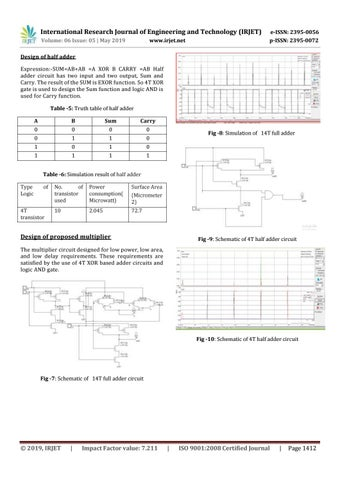International Research Journal of Engineering and Technology (IRJET)

e-ISSN: 2395-0056

Volume: 06 Issue: 05 | May 2019

p-ISSN: 2395-0072

www.irjet.net

Design of half adder Expression:-SUM=AB+AB =A XOR B CARRY =AB Half adder circuit has two input and two output, Sum and Carry. The result of the SUM is EXOR function. So 4T XOR gate is used to design the Sum function and logic AND is used for Carry function. Table -5: Truth table of half adder A

B

Sum

Carry

0

0

0

0

0

1

1

0

1

0

1

0

1

1

1

1

Fig -8: Simulation of 14T full adder

Table -6: Simulation result of half adder Type Logic

of

4T transistor

No. of transistor used

Power consumption( Microwatt)

Surface Area (Micrometer 2)

10

2.045

72.7

Design of proposed multiplier

Fig -9: Schematic of 4T half adder circuit

The multiplier circuit designed for low power, low area, and low delay requirements. These requirements are satisfied by the use of 4T XOR based adder circuits and logic AND gate.

Fig -10: Schematic of 4T half adder circuit

Fig -7: Schematic of 14T full adder circuit

ÂŠ 2019, IRJET

|

Impact Factor value: 7.211

|

ISO 9001:2008 Certified Journal

|

Page 1412

# IRJET- Dadda Algorithm based Lowpower High Speed Multiplier using 4T XOR Gate

https://www.irjet.net/archives/V6/i5/IRJET-V6I5282.pdf

# IRJET- Dadda Algorithm based Lowpower High Speed Multiplier using 4T XOR Gate

https://www.irjet.net/archives/V6/i5/IRJET-V6I5282.pdf